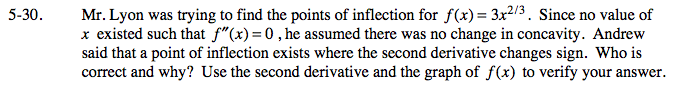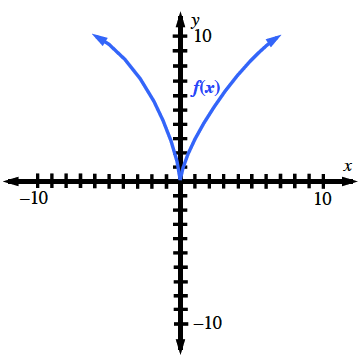### Home > CALC > Chapter 5 > Lesson 5.1.3 > Problem5-30

5-30.

Mr. Lyon was trying to find the points of inflection for f(x) = 3x2/3. Since no value of x existed such that f ′′(x) = 0, he assumed there was no change in concavity. Andrew said that a point of inflection exists where the second derivative changes sign. Who is correct and why? Use the second derivative and the graph of f(x) to verify your answer. Homework Help ✎CANDIDATES for points of inflection can be found where f ''(x) = 0 and where f ''(x) = DNE. You must check both.Notice that there is not a concavity change at the cusp.